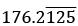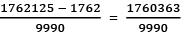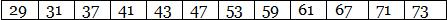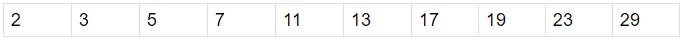Courses

# Arun Sharma Test: Number System- 2

## 15 Questions MCQ Test Quantitative Aptitude (Quant) | Arun Sharma Test: Number System- 2

Description
This mock test of Arun Sharma Test: Number System- 2 for CAT helps you for every CAT entrance exam. This contains 15 Multiple Choice Questions for CAT Arun Sharma Test: Number System- 2 (mcq) to study with solutions a complete question bank. The solved questions answers in this Arun Sharma Test: Number System- 2 quiz give you a good mix of easy questions and tough questions. CAT students definitely take this Arun Sharma Test: Number System- 2 exercise for a better result in the exam. You can find other Arun Sharma Test: Number System- 2 extra questions, long questions & short questions for CAT on EduRev as well by searching above.
QUESTION: 1

### What is the difference between the Place Value and Face Value of 9 in 798652?

Solution:

► Face Value of any number remains the Same irrespective of the place, for 9 it is 9
► Place Value = Place Multiplier x  Face Value
⇒ 10000 x 9
⇒ 90000
► Place Value - Face Value = 90000 - 9 = 89991

QUESTION: 2

### Convertin p/q form

Solution:

► All the digits written once = 1762125
► All the digits without Bar written once = 1762
► No. of digits with bar after decimal = 3
► No. of digits without bar after decimal = 1
Rational form =QUESTION: 3

### (a2 + 2ab + b2) will fall under which of the categories, a and b are Integers

Solution:

► There are 4 case in the same
► Case 1 : a and b both are odd

⇒ a2 + 2ab + b2
⇒ Odd2 + 2 x Odd x Odd +Odd2
⇒ Odd + Even + Odd
⇒ Even

Case 2 : a and b both are even

⇒ a2 + 2ab + b2
⇒ Even2 + 2 × Even × Even + Even2
⇒ Even + Even + Even
⇒ Even

► Case 3 : a is odd and b is even

⇒ a2 + 2ab + b2
⇒ Odd2 + 2 x Odd x Even + Even2
⇒ Odd + Even + Even
⇒ Odd

Case 4 : a is even and b is odd

⇒ a2 + 2ab + b2
⇒ Even2 + 2 × Even × Odd + Odd2
⇒ Even + Even + Odd
⇒ Odd

► In 2 Cases its Odd and in other 2 it is Even, so we cannot say anything about the answer.

QUESTION: 4

If (6a + 12) is odd then 'a' would be, a is an Integer

Solution:

► There are two possibilities for a
► Case 1: a is even

⇒ 6a  = Even x Even = Even
⇒ 6a + 12 = Even + Even = Even

► Case 2: a is odd

⇒ 6a = Even x Odd = Even
⇒ 6a + 12 = Even + Even = Even

► In both the cases the answer is even, so the information provided is inconsistent.

QUESTION: 5

(abc) is odd what would (a2 + b2 + c2) be, a, b and c are Integers.

Solution:

► For abc to be Odd the only case possible is none of them being Even i.e. a, b and c all three are Odd

⇒ a2 + b2 + c2
⇒ Odd2 + Odd2 + Odd2
⇒ Odd + Odd + Odd
⇒ Odd

QUESTION: 6

What is the smallest positive Even Rational Number?

Solution:

► Option A : 0, It is even and rational but not positive
► Option B : 1, it is positive and rational but odd
► Option C : 2, it is even positive and rational. Also the smallest
► Option D : 4, it is even, positive and rational but not the smallest

QUESTION: 7

How many prime numbers are there between 25 and 75?

Solution:

► There are 12 prime numbers between 25 and 75 as listed below:QUESTION: 8

Which is the largest Even Prime number?

Solution:

► There is only one Even prime number i.e. 2.

QUESTION: 9

Which of the following is a prime number?

Solution:

► We know the property that a prime number when divided by 6 gives a remainder of 1 or 5.
► Option A : 8831 divided by 6 gives a remainder of 5
► Option B : 8829 divided by 6 gives a remainder of 3
► Option C : 8833 divided by 6 gives a remainder of 1, but is divisible by 11
► Option D : 8835 divided by 6 gives a remainder of 3

QUESTION: 10

If a and b are odd numbers, which of the following is surely even?

Solution:

► Option A : a2 + b2 = Odd2 + Odd2 = Odd + Odd = Even
► Option B  : a2 + 2b2 = Odd2 + Even × Odd2 = Odd + Even = Odd
► Option C ∶ 2a2 + b2 = Even × Odd2 + Odd2 = Even + odd = Odd
► Option D ∶ a2 + b2 + ab = Odd2 + Odd2 + Odd × Odd = Odd + Odd + Odd = Odd

QUESTION: 11

Sum of any 50 consecutive 4 digit Whole numbers would always be:

Solution:

► Sum of Any Consecutive 50 Whole Numbers

⇒ Sum of 25 Even Numbers + Sum of 25 Odd Numbers
⇒ Even + Odd
⇒ Odd

QUESTION: 12

The sum of first 10 Prime numbers is

Solution:

► First 10 Prime numbers are listed Below :► Their sum = 129

QUESTION: 13

How many Prime numbers less than 1000 are divisible by 17?

Solution:

► Being Prime numbers any number would be divisible by 1 or itself.
► Since 17 is a prime number. It will be the only prime number less than 1000 which will be divisible by 17.

QUESTION: 14

How many Prime numbers less than 1000 are divisible by 16?

Solution:

► Being Prime numbers any number would be divisible by 1 or itself.
► Since 16 is not a prime number. There would be no other prime number divisible by 16.

QUESTION: 15

How many pairs of consecutive odd prime numbers are there less than 200?

Solution:

► The List of such pairs is listed below:
(3,5); (5,7); (11,13); (17,19); (29,31); (41,43); (59,61); (71,73), (101,103); (107,109); (137,139);  (149,151); (179,181); (191,193); (197,199)

► ​15 Such pairs exist.